# 6. Virtual Implementation - B-Division-2022-2023-Odd/Repo-02 GitHub Wiki

INTEGRATED UNIT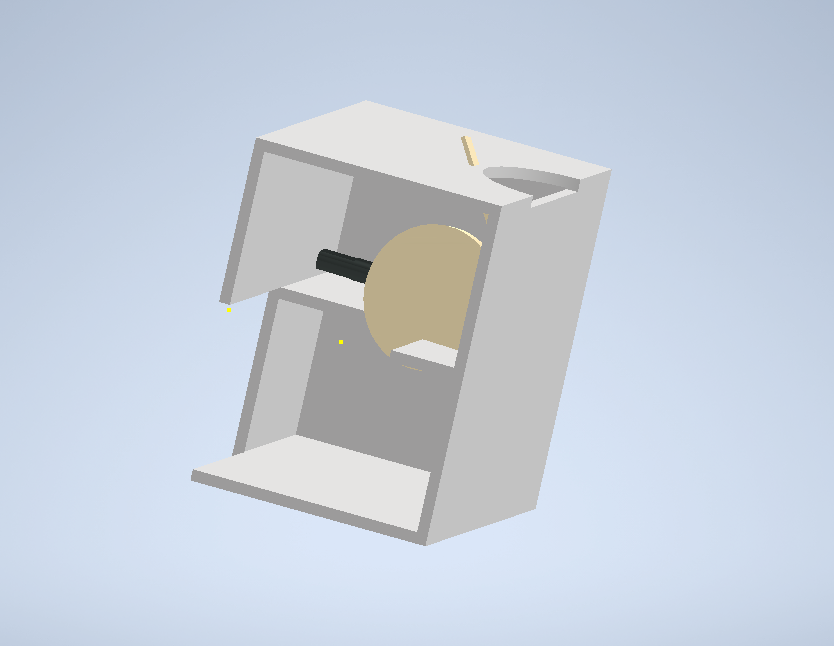HOUSING UNIT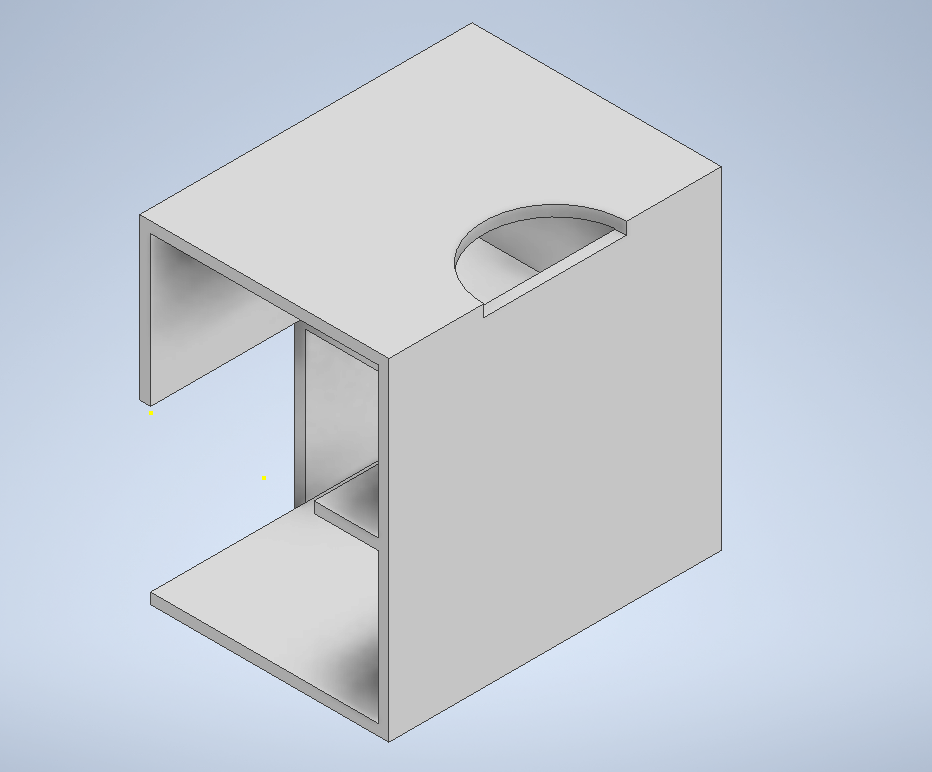MECHANISM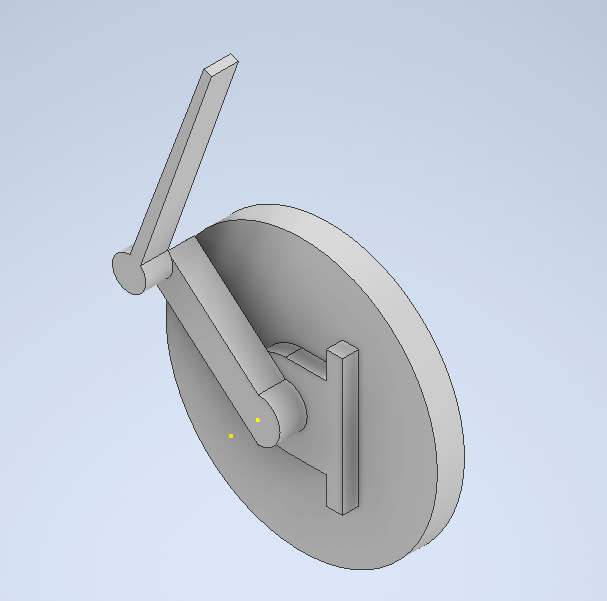MOTION UNIT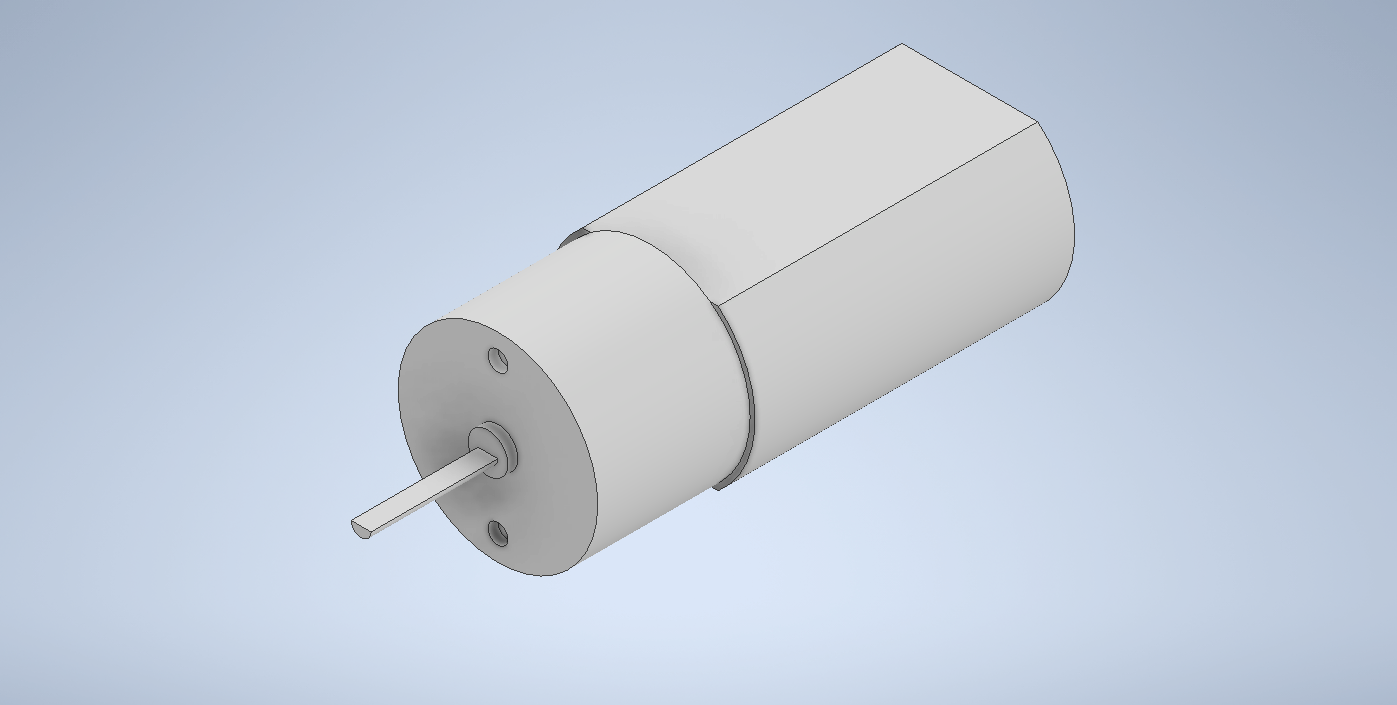CIRCUIT DIAGRAM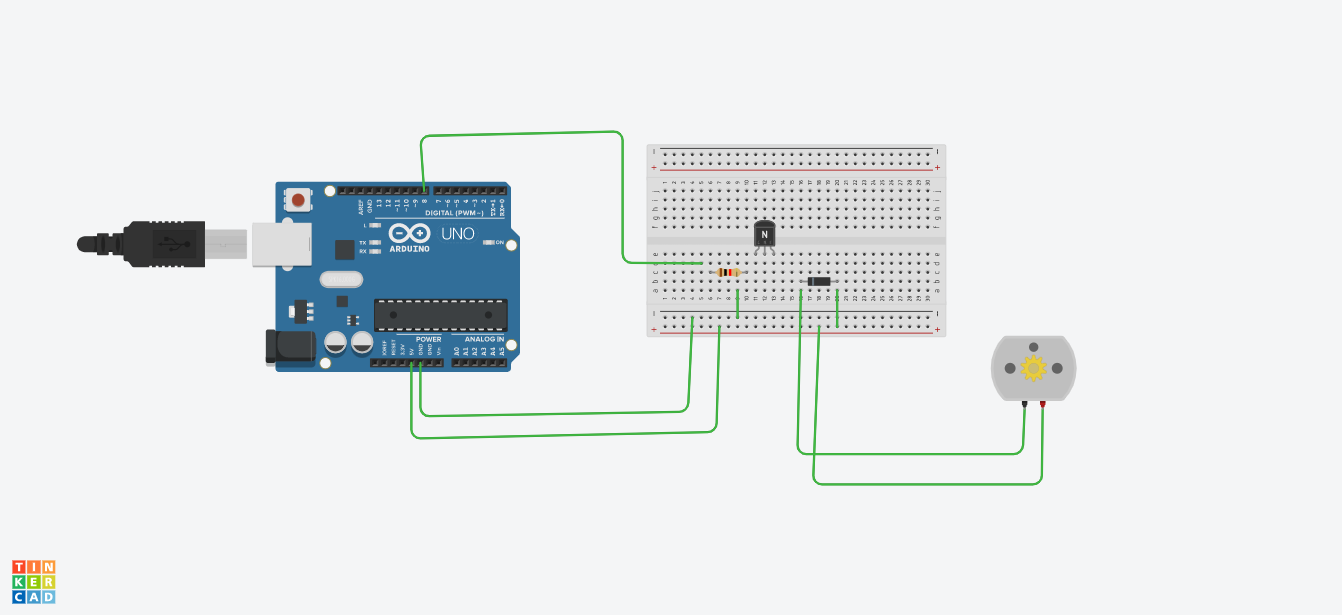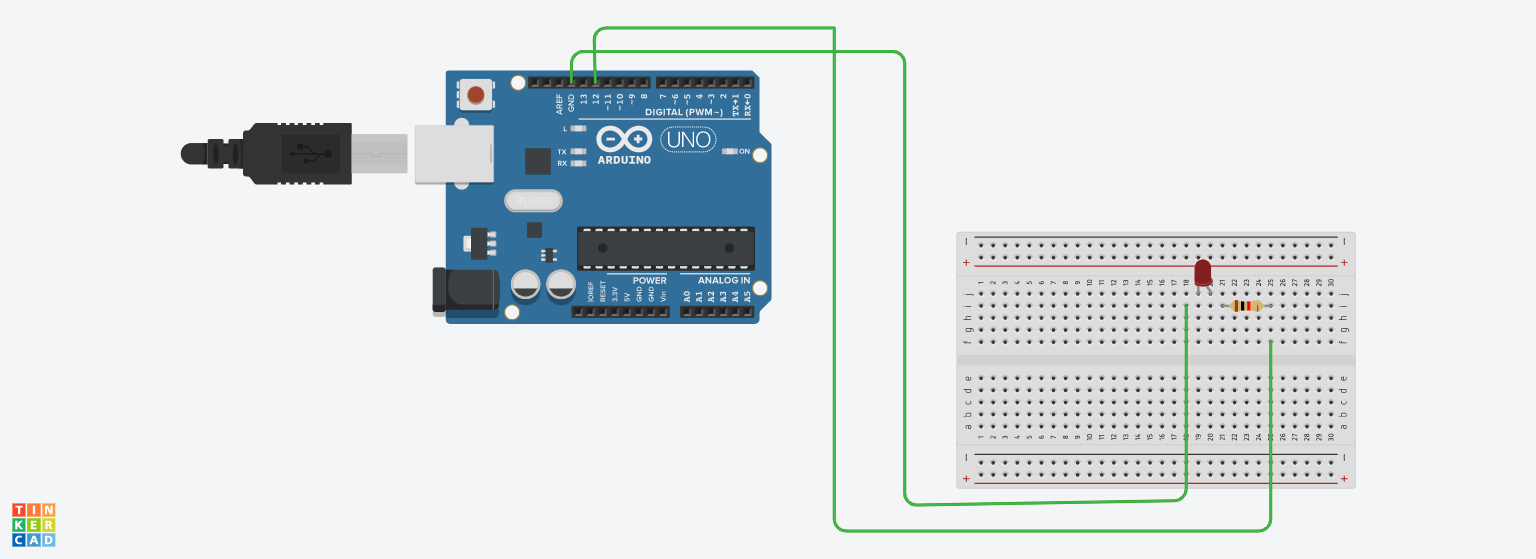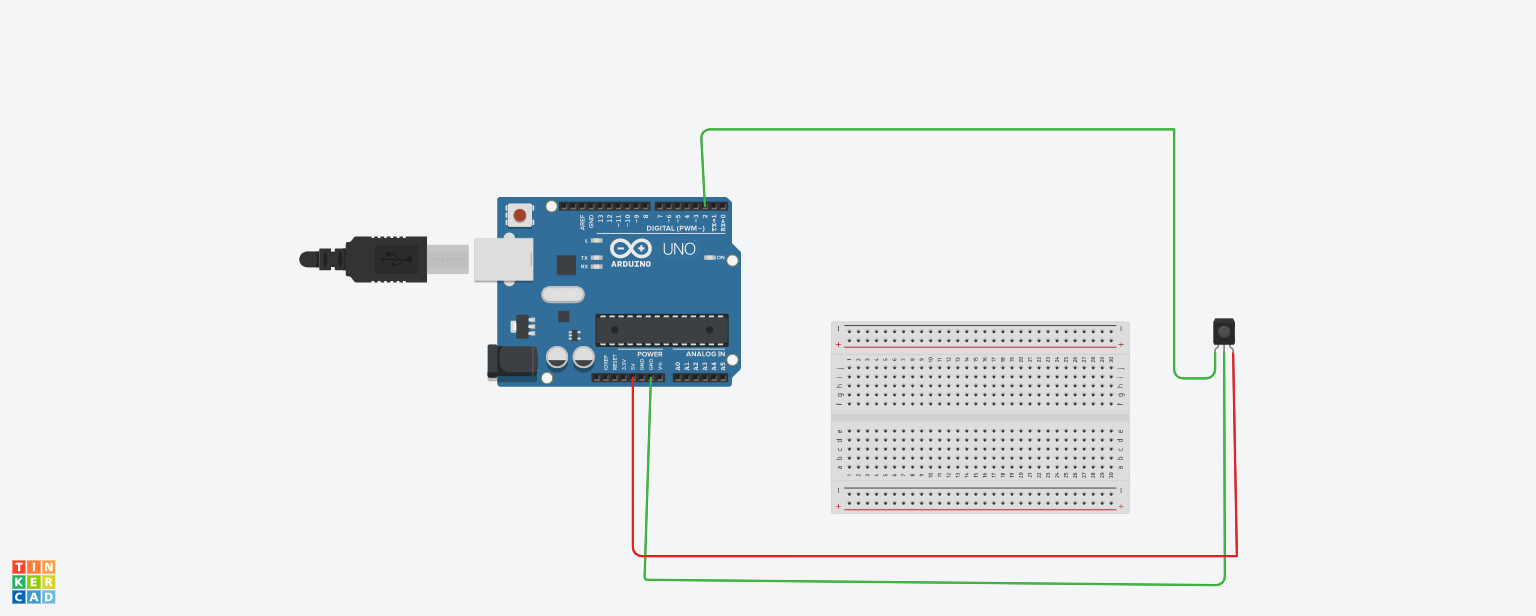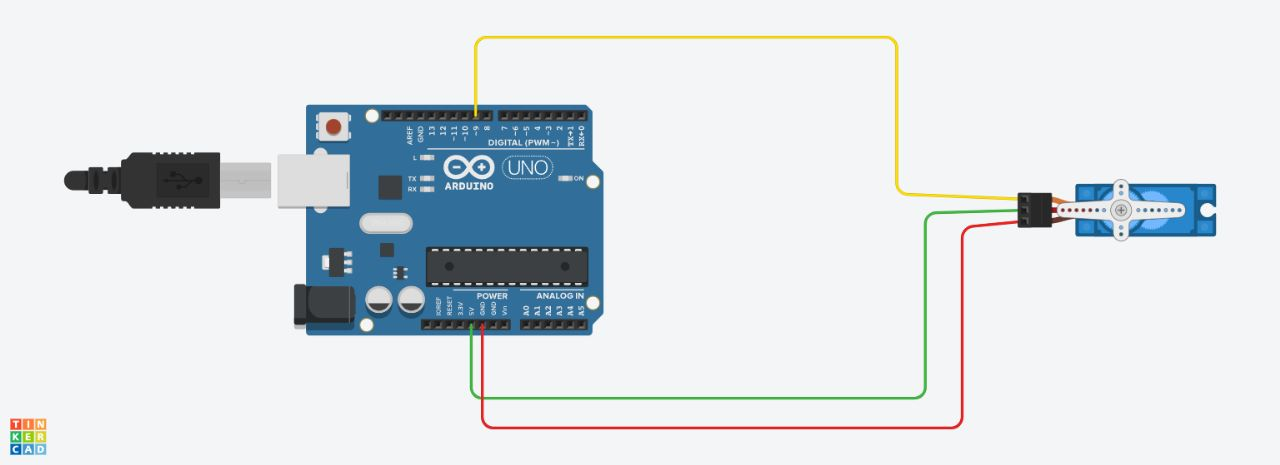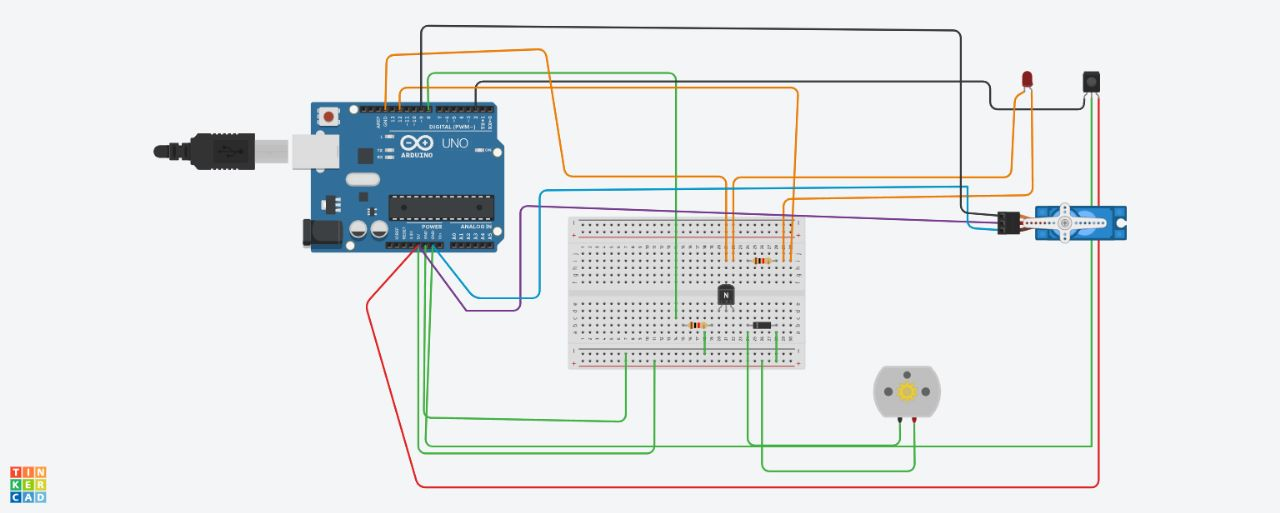FLOWCHART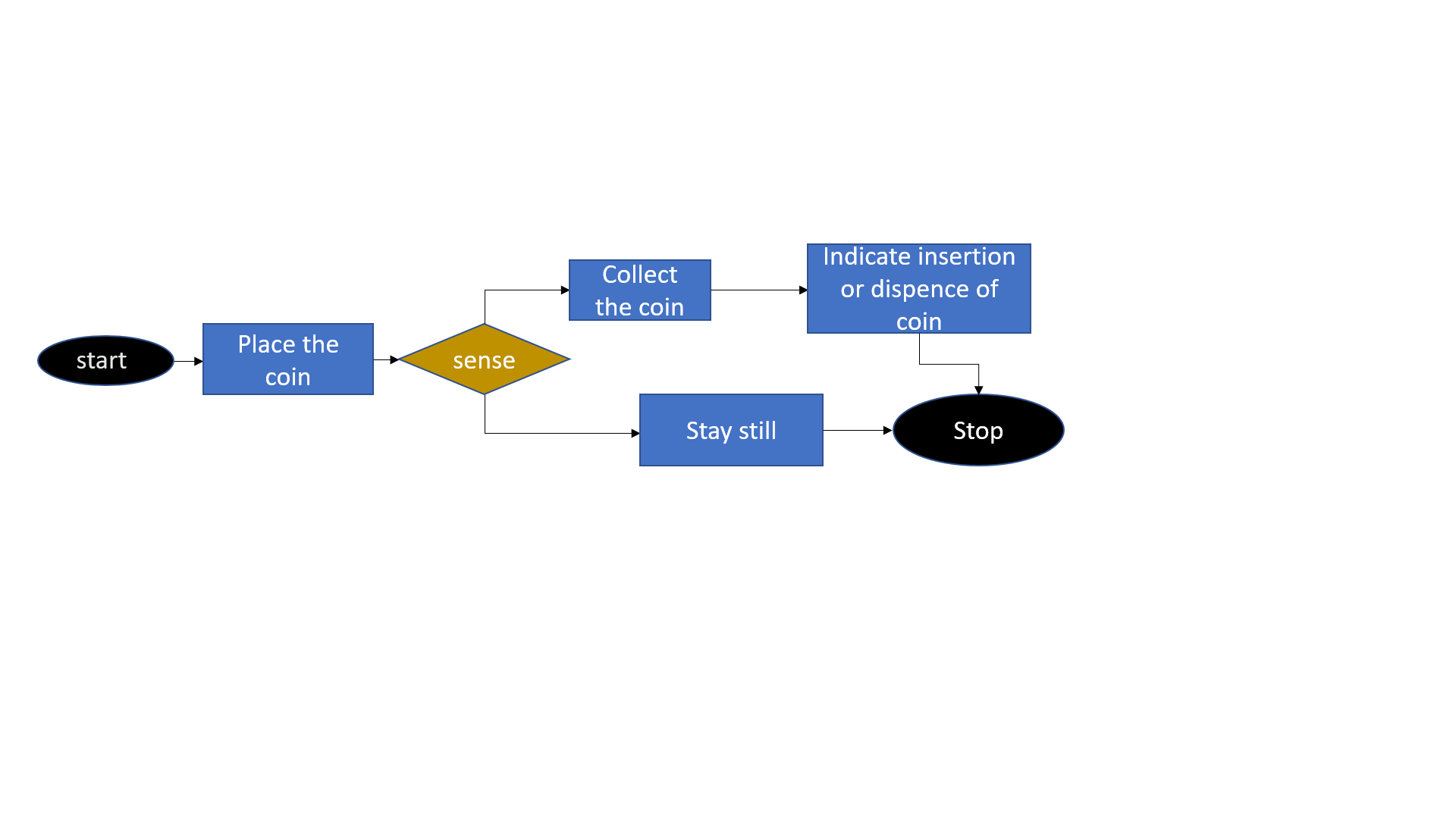# Bill of materials

Sl.no. Part name Material used Description Quantity
1. Housing Box TOP foam board 0.75ft * 0.75ft * 10mm thickness 1
2. Housing Box Sides foam board 1ft * 0.75ft * 10mm thickness 4
3. Housing Box Bottom foam board 0.75ft * 0.75ft * 10mm thickness 1
4. Microcontroller Arduino ATMEGA2560 1
5. L Clamps - 0.5 in 16
6. Hinges - 1.5 in 4
7. Actuators DC Motors 1
8. Screw and Washer - diameter 8mm & length 20mm
9. Sensor IR sensor - 1
10. LED Bulb - red color 1
11. Buzzer Electronic Buzzer 22.5mm diameter 1
12. Screws -
13. Jumper Wires - - 14
14. Stand for DC Motor foam board 1
15. Storage Box foam board 120mm * 80mm * 60mm * 5mm thickness 1
16. gears Acrylic 8

MOTOR SIZING

1. VOLUME OF MECHANISM

MAIN ROD L= 7.06cm , B=1.56cm , H=.5cm volume=5.5068 mm^3

SECONDARY ROD L=9.652cm , B=.43cm , H=.5cm volume=2.0752 cm^3

MAIN CIRCLE DIAMETER=12.7cm , thickness=.5cm 6.35 cm^3

TOTAL VOLUME=13.932 cm^3

density of acrylic sheet=1.18 gm/cm^3

MASS OF MECHANISM= 13.932 x 1.18= 16.4398 gm

1. VOLUME OF MONSTER

L=3cm, H=6cm, B=4cm

Volume= LxBxH = 3x6x4 = 72 cm^3 VOLUME OF MONSTER:

Density of Plastic= 0.92 g/cm^3

mass = 72 x 0.92 = 66.24 g

1. MASS OF COINS

mass of coins= 5g

1. TOTAL MASS

Total mass = 16.4398 + 66.24 + 5 = 87.6798 gm = 0.08768 kg

5.Force involved is Vertical Force which is given by the formula

F = m x g F = 0.08768 x 9.8 F = 0.8593

6.Torque

T= F x r T= 0.8593 x 2.5 T= 2.15 BATTERY SIZING load current=250mA IR sensor load current=20mA Total current=270mA SOF1.2=324mA Therefore, Required adapter is 5v&0.5A